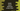# Check odd or even using 'when' in Kotlin# Introduction :

We can always find if a number is odd or even is to use one if-else block. This is the easiest process used in all programming languages. But in Kotlin, we can also use one when block. when block is more powerful. You can use it as a statement or as an expression. statement means it will work as an if-else statement and expression mean it will return value based on an input.

Now, to check if a number is odd or even using a when block, we will take the number as an input from the user. The user will enter the number, it will check and verify if it is odd or even and print out the output :

## Kotlin program :

``````import java.util.*

fun main() {
val scanner = Scanner(System.`in`)
print("Enter a number : ")

val number = scanner.nextInt()

when {
number % 2 == 0 -> println("\$number is even")
number % 2 != 0 -> println("\$number is odd")
}
}
``````

Here, we are using one Scanner object to read the user input number. nextInt() is used for that. Next, the when block is checking if the input number is odd or even.

Inside the when block, we have two statements. It will run both statements one by one. You can also use else for the second statement instead of checking the condition again :

``````import java.util.*

fun main() {
val scanner = Scanner(System.`in`)
print("Enter a number : ")

val number = scanner.nextInt()

when {
number % 2 == 0 -> println("\$number is even")
else -> println("\$number is odd")
}
}
``````

### Sample Output :

``````Enter a number : 12
12 is even

Enter a number : 13
13 is odd
``````

You can also add more statements to a when block. Try to run it and drop one comment below if you have any queries.

### Using when as expression :

You can also store the result in a different variable for later use like below :

``````import java.util.*

fun main() {
val scanner = Scanner(System.`in`)
print("Enter a number : ")

val number = scanner.nextInt()

val result = when {
number % 2 == 0 -> "\$number is even"
else -> "\$number is odd"
}
println(result)
}
``````

Use this approach if you want to store the result value in a different variable.